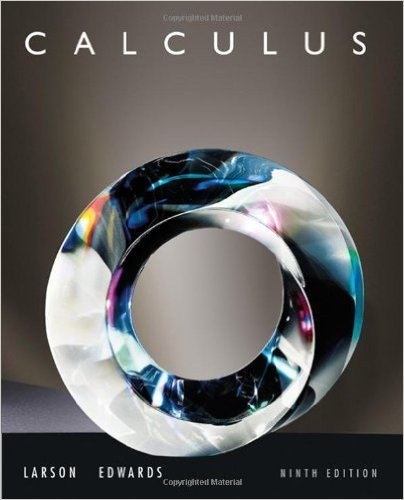×
×

# Solutions for Chapter 8.2: Integration by Parts## Full solutions for Calculus | 9th Edition

ISBN: 9780547167022Solutions for Chapter 8.2: Integration by Parts

Solutions for Chapter 8.2
4 5 0 280 Reviews
25
5
##### ISBN: 9780547167022

This textbook survival guide was created for the textbook: Calculus , edition: 9. Calculus was written by and is associated to the ISBN: 9780547167022. This expansive textbook survival guide covers the following chapters and their solutions. Chapter 8.2: Integration by Parts includes 120 full step-by-step solutions. Since 120 problems in chapter 8.2: Integration by Parts have been answered, more than 63891 students have viewed full step-by-step solutions from this chapter.

Key Calculus Terms and definitions covered in this textbook

If u = v and w = z , then u + w = v + z

• Ambiguous case

The case in which two sides and a nonincluded angle can determine two different triangles

• Aphelion

The farthest point from the Sun in a planet’s orbit

• artesian coordinate system

An association between the points in a plane and ordered pairs of real numbers; or an association between the points in three-dimensional space and ordered triples of real numbers

• Compound interest

Interest that becomes part of the investment

• Definite integral

The definite integral of the function ƒ over [a,b] is Lbaƒ(x) dx = limn: q ani=1 ƒ(xi) ¢x provided the limit of the Riemann sums exists

• Descriptive statistics

The gathering and processing of numerical information

• Fitting a line or curve to data

Finding a line or curve that comes close to passing through all the points in a scatter plot.

• Increasing on an interval

A function ƒ is increasing on an interval I if, for any two points in I, a positive change in x results in a positive change in.

• Measure of an angle

The number of degrees or radians in an angle

• Multiplicative inverse of a real number

The reciprocal of b, or 1/b, b Z 0

• Natural numbers

The numbers 1, 2, 3, . . . ,.

• NDER ƒ(a)

See Numerical derivative of ƒ at x = a.

• Numerical derivative of ƒ at a

NDER f(a) = ƒ1a + 0.0012 - ƒ1a - 0.00120.002

• Open interval

An interval that does not include its endpoints.

• Product of matrices A and B

The matrix in which each entry is obtained by multiplying the entries of a row of A by the corresponding entries of a column of B and then adding

• Secant

The function y = sec x.

• Sum of two vectors

<u1, u2> + <v1, v2> = <u1 + v1, u2 + v2> <u1 + v1, u2 + v2, u3 + v3>

• Velocity

A vector that specifies the motion of an object in terms of its speed and direction.

• Vertices of a hyperbola

The points where a hyperbola intersects the line containing its foci.

×